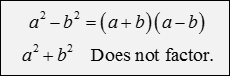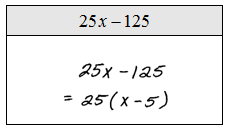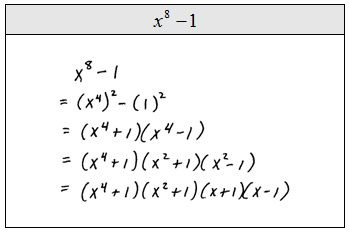## Algebra

Showing posts with label general guidelines. Show all posts
Showing posts with label general guidelines. Show all posts

### General Guidelines for Factoring Polynomials

Now that we have learned techniques for factoring 4, 3, and 2-term polynomials, we are ready to practice by mixing up the problems. The challenge is to first identify the type of factoring problem then decide which method to apply.

The basic guidelines for factoring follow:
1. Look to factor out any GCF.
2. Four-Term Polynomials - Factor by grouping.
3. Trinomials - Factor using the "guess and check" method.
4. Binomials - Use the the special products in this order:
Sum and Difference of SquaresSum and Difference of Cubes* If a binomial is both a difference of squares and cubes, then to obtain a more complete factorization, factor it as a difference of squares first.
* Not all polynomials factor.  In this case, beginning algebra students may write, "does not factor - DNF."

Factor.Tip: Make some note cards to aid in helping memorize the formulas for the special products. Look for factors to factor further - sometimes factoring once is not enough.

Factor.Take some time to understand the difference between the last two solved problems. Notice that x^6 - y^6  is both a difference of squares and a difference of cubes at the same time. Here we chose to apply the difference of squares formula first. On the other hand, for x^6 + y^6 we chose to apply the sum of cubes formula first because it does not factor as a sum of squares.

Factor.Video Examples on YouTube: Factor the following polynomials.